Select Page

# Principle of Electromagnetic Energy Conversion

Principle of Electromagnetic Energy Conversion

Contents

Recall the terms: Magnet, Magnetic Field, Magnetic Lines of Forces, Magnetic Flux, and Magnetic Flux Density 1

Recall the terms: MMF, Magnetic Field Strength, Permeability, Reluctance, and Permeance 3

Describe Magnetic Circuits and their types 5

Recall the following terms i. Flux Fringing ii. Leakage Flux iii. Leakage Coefficient 7

Recall the following Laws/Rules: Ampere’s Circuital Law 11

Recall the following Laws/Rules: Right-Hand Thumb Rule as per Electrical machines 12

Recall the following Laws/Rules: Fleming’s Left-Hand Rule 12

Recall the following Laws/Rules: Faraday’s Law of Electromagnetic Induction and Lenz’s Law 14

Recall the following Laws/Rules: Fleming’s Right-Hand Rule 16

Recall the principle of Electromechanical Energy Conversion 18

Recall the concept of Energy and Co-energy 20

Describe the Magnetic Energy stored in Singly Excited Systems 21

Describe the Torque developed in Doubly Excited Systems 22

# Recall the terms: Magnet, Magnetic Field, Magnetic Lines of Forces, Magnetic Flux, and Magnetic Flux Density

1. Magnet:

A magnet is a material or object that produces a magnetic field, which is a force that can attract or repel certain materials. Magnets can be found in various shapes, such as bars, disks, horseshoes, and rings. They are commonly made from materials such as iron, nickel, and cobalt.

Example: A refrigerator magnet that holds a picture or note to the door.

1. Magnetic Field:

A magnetic field is a region in space where a magnetic force can be detected. It is created by the motion of electric charges, such as electrons, within a material or a current-carrying conductor. The direction and strength of the magnetic field are determined by the direction and intensity of the current or the magnetic material.

Example: The Earth has a magnetic field that is generated by the motion of molten iron in its core.

1. Magnetic Lines of Force:

Magnetic lines of force are imaginary lines that depict the direction and strength of a magnetic field. The direction of the magnetic field at any point is represented by the tangent to the line of force at that point. The closer the lines of force are, the stronger the magnetic field.

Example: Iron filings sprinkled around a bar magnet form patterns that depict the magnetic lines of force.

1. Magnetic Flux:

Magnetic flux is the measure of the total magnetic field that passes through a given area. It is represented by the symbol Φ and is measured in webers (Wb). The magnetic flux is proportional to the strength of the magnetic field and the area through which it passes.

Example: A coil of wire that is placed in a magnetic field will generate an electrical current that is proportional to the magnetic flux passing through the coil.

1. Magnetic Flux Density:

Magnetic flux density is the measure of the magnetic field strength at a particular point in space. It is represented by the symbol B and is measured in teslas (T). The magnetic flux density is proportional to the strength of the magnetic field and is inversely proportional to the distance from the source of the field.

Example: The magnetic flux density of a permanent magnet can be measured using a teslameter.

# Recall the terms: MMF, Magnetic Field Strength, Permeability, Reluctance, and Permeance

1. MMF:

MMF stands for Magnetomotive force, which is the measure of the energy or force that drives magnetic flux through a magnetic circuit. It is represented by the symbol F and is measured in ampere-turns. MMF is generated by the flow of electric current through a coil of wire or a magnetic material.

Example: The MMF of a solenoid is calculated by multiplying the number of turns in the coil by the current flowing through it.

1. Magnetic Field Strength:

Magnetic field strength is the measure of the intensity of a magnetic field. It is represented by the symbol H and is measured in amperes per meter (A/m). The magnetic field strength is proportional to the MMF and inversely proportional to the length of the magnetic path.

Example: The magnetic field strength at a point in a magnetic circuit can be calculated by dividing the MMF by the length of the magnetic path.

1. Permeability:

Permeability is the measure of a material’s ability to conduct magnetic flux. It is represented by the symbol μ and is measured in henries per meter (H/m). The permeability of a material depends on its composition, temperature, and magnetic history.

Example: Iron has a higher permeability than air, which makes it a better material for creating magnetic circuits.

1. Reluctance:

Reluctance is the measure of a material’s opposition to magnetic flux. It is represented by the symbol R and is measured in ampere-turns per weber (AT/Wb). The reluctance of a material depends on its composition, shape, and dimensions.

Example: The reluctance of a magnetic circuit can be calculated by dividing the length of the magnetic path by the permeability and cross-sectional area of the material.

1. Permeance:

Permeance is the measure of a material’s ability to conduct magnetic flux. It is the reciprocal of reluctance and is represented by the symbol P. It is measured in webers per ampere-turn (Wb/AT). The permeance of a material depends on its composition, shape, and dimensions.

Example: The permeance of a magnetic circuit can be calculated by dividing the permeability and cross-sectional area of the material by the length of the magnetic path.

# Describe Magnetic Circuits and their types

A magnetic circuit is a closed path along which a magnetic flux flows. It is analogous to an electric circuit, where the current flows along a closed path. In a magnetic circuit, the magnetic flux is generated by a source, such as a magnet or an electric current, and flows through a material with a certain permeability, such as iron or steel.

There are two main types of magnetic circuits:

1. Series Magnetic Circuit:

In a series magnetic circuit, the magnetic flux flows through a series of magnetic materials that are connected end-to-end. The magnetic flux is the same through each material, and the total magnetic flux is equal to the sum of the fluxes through each material. The reluctance of the circuit is the sum of the reluctances of each material.

Example: A solenoid, where the magnetic flux flows through the core and the air gap, which are connected in series.

1. Parallel Magnetic Circuit:

In a parallel magnetic circuit, the magnetic flux is split into multiple paths, each flowing through a separate magnetic material. The total magnetic flux is equal to the sum of the fluxes through each path. The reluctance of the circuit is the reciprocal of the sum of the reciprocals of the reluctances of each path.

Example: An electromagnet, where the magnetic flux flows through the core and the yoke, which are connected in parallel.

Magnetic circuits can also be classified based on their shape and size, such as:

1. Straight Magnetic Circuit:

A straight magnetic circuit is a circuit that has a straight path for the magnetic flux to flow. It is commonly used in applications such as solenoids and transformers.

1. Cylindrical Magnetic Circuit:

A cylindrical magnetic circuit is a circuit that has a cylindrical shape, such as a motor or a generator.

1. Annular Magnetic Circuit:

An annular magnetic circuit is a circuit that has a ring-shaped path for the magnetic flux to flow, such as a transformer.

In conclusion, magnetic circuits are essential components in various electrical and electronic devices that require the control and manipulation of magnetic fields. Understanding the different types of magnetic circuits and their properties is crucial in designing and analyzing these devices.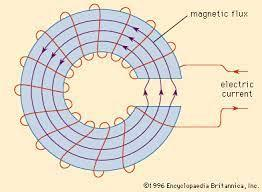# Recall the following terms i. Flux Fringing ii. Leakage Flux iii. Leakage Coefficient

1. Flux Fringing:

Flux fringing is a phenomenon that occurs at the edges of a magnetic material or air gap in a magnetic circuit. The magnetic field lines become distorted, causing the magnetic flux to leak out of the main path and flow through the surrounding air. This can result in a decrease in the overall magnetic flux in the circuit and can affect the performance of the device.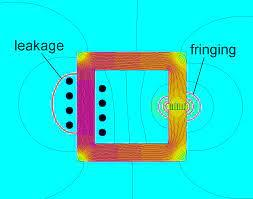The formula for flux fringing can be approximated using the following equation:

ΔB = (μ * I)/(2πr)

where ΔB is the change in flux density due to fringing, μ is the permeability of the material, I is the current flowing through the component, and r is the radius of the component.

Example: In a transformer, the flux fringing can cause a loss of magnetic flux and reduce the efficiency of the transformer.

1. Leakage Flux:

Leakage flux is the magnetic flux that does not follow the main path in a magnetic circuit and flows through adjacent materials or air gaps. This can occur due to flux fringing, non-uniform material properties, and geometric irregularities. Leakage flux can cause a decrease in the efficiency of the device and can cause electromagnetic interference.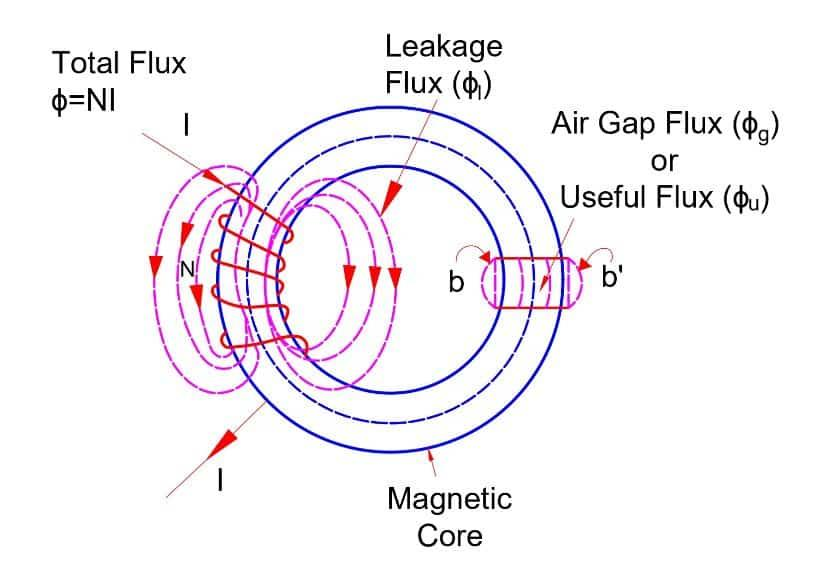The formula for leakage flux can be approximated using the following equation:

Φleak = Φtotal – Φcoupled

where Φtotal is the total magnetic flux produced by the primary winding of a transformer or the current-carrying conductor of an inductor, and Φcoupled is the magnetic flux that couples between the primary and secondary windings of a transformer or between the conductor and the magnetic core of an inductor.

Example: In a motor, the leakage flux can cause eddy currents in nearby conductive materials, leading to unwanted heating and loss of energy.

1. Leakage Coefficient:

The leakage coefficient is a measure of the leakage flux in a magnetic circuit, represented by the symbol k. It is defined as the ratio of the leakage flux to the total flux in the circuit. A higher leakage coefficient indicates a higher proportion of leakage flux and can result in decreased performance and efficiency of the device.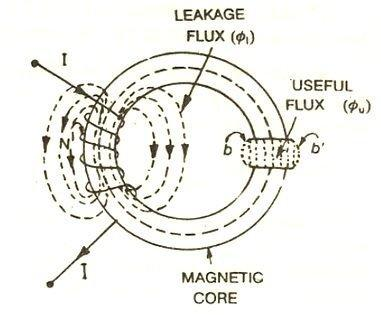The formula for leakage coefficient can be expressed as:

k = Φleaktotal

where k is the leakage coefficient, Φleak is the amount of leakage flux, and Φtotal is the total magnetic flux produced by the component.

Example: The leakage coefficient of a transformer can be calculated by measuring the total flux and the leakage flux and dividing the latter by the former.

In conclusion, understanding the concepts of flux fringing, leakage flux, and leakage coefficient is crucial in the design and analysis of magnetic circuits and devices. These phenomena can significantly impact the performance and efficiency of the device and need to be taken into consideration during the design process.

# Recall the following Laws/Rules: Ampere’s Circuital Law

Ampere’s circuital law is a fundamental principle in electromagnetism that relates the magnetic field to the electric current flowing in a closed loop. The law states that the line integral of the magnetic field around a closed loop is equal to the product of the electric current passing through the loop and the permeability of the medium in which the current is flowing. Mathematically, the law can be written as:

∮B·dl = μ0·I

where ∮B·dl is the line integral of the magnetic field B around a closed loop, I is the electric current passing through the loop, and μ0 is the permeability of free space.

Ampere’s law is a powerful tool for calculating the magnetic field around a current-carrying conductor or a magnetic circuit. It is particularly useful in cases where the geometry of the system is symmetric, and the current density is uniform.

Example: Consider a long straight wire carrying a steady current I. According to Ampere’s law, the line integral of the magnetic field B around a circular loop of radius r centred on the wire is equal to μ0·I, where μ0 is the permeability of free space. The magnetic field B can be calculated using the formula B = μ0·I/2πr, which shows that the magnetic field decreases with increasing distance from the wire.

Ampere’s law can also be used to calculate the magnetic field inside a solenoid, where the current flows in a closed loop along the length of the solenoid. In this case, the magnetic field inside the solenoid is uniform and proportional to the current density.

In conclusion, Ampere’s circuital law is a fundamental principle in electromagnetism that relates the magnetic field to the electric current flowing in a closed loop. The law has many applications in the calculation and design of magnetic circuits and devices.

# Recall the following Laws/Rules: Right-Hand Thumb Rule as per Electrical machines

The Right-Hand Thumb Rule is widely used in electrical machines to determine the direction of the magnetic field, the direction of the current flow, and the direction of the force exerted on a conductor in a magnetic field. Here are a few examples of how the rule is applied in electrical machines:

1. Direction of Magnetic Field: When a current-carrying conductor is placed in a magnetic field, the magnetic field around the conductor is perpendicular to the direction of the current flow. To determine the direction of the magnetic field, the Right-Hand Thumb Rule is used. If the right-hand thumb points in the direction of the current flow, then the curled fingers of the right hand will show the direction of the magnetic field around the conductor.
2. Direction of Current Flow: The Right-Hand Thumb Rule can also be used to determine the direction of current flow in a conductor. If the direction of the magnetic field is known, the right-hand thumb can be used to point in the direction of the magnetic field. The curled fingers of the right hand will then show the direction of the current flow.
3. Direction of Force: The Right-Hand Thumb Rule can also be used to determine the direction of force exerted on a current-carrying conductor in a magnetic field. If the direction of the current flow and the magnetic field is known, the right-hand thumb can be used to point in the direction of the current flow. The curled fingers of the right hand will then show the direction of the force exerted on the conductor.

In conclusion, the Right-Hand Thumb Rule is an important tool for understanding the behavior of electrical machines, as it can be used to determine the direction of the magnetic field, the direction of the current flow, and the direction of the force exerted on a conductor in a magnetic field.

# Recall the following Laws/Rules: Fleming’s Left-Hand Rule

Fleming’s left-hand rule is a tool for determining the direction of the force experienced by a current-carrying conductor in a magnetic field. The rule is based on the relationship between the magnetic field, electric current, and the force experienced by the conductor, as described by the Lorentz force law.

The rule is used by holding the left hand in a particular way, with the thumb, index finger, and middle finger all pointing in different directions, representing the direction of the magnetic field, current, and force, respectively.

Example: Consider a current-carrying wire placed in a magnetic field. Using Fleming’s left-hand rule, we can determine the direction of the force experienced by the wire. If we point the index finger of our left hand in the direction of the magnetic field, the middle finger in the direction of the current flow, the thumb will point in the direction of the force experienced by the wire. For example, if the magnetic field is pointing in the positive z-direction, the current is flowing in the positive x-direction, the force will be in the positive y-direction.

Fleming’s left-hand rule is also useful in determining the direction of the force on a charged particle moving in a magnetic field. The force experienced by a charged particle is perpendicular to both the magnetic field and the velocity of the particle.

Example: Consider a positively charged particle moving in a magnetic field. Using Fleming’s left-hand rule, we can determine the direction of the force experienced by the particle. If we point the index finger of our left hand in the direction of the magnetic field, the middle finger in the direction of the velocity of the particle, the thumb will point in the direction of the force experienced by the particle. For example, if the magnetic field is pointing in the positive z-direction, and the particle is moving in the positive x-direction, the force will be in the positive y-direction.

In conclusion, Fleming’s left-hand rule is a tool for determining the direction of the force experienced by a current-carrying conductor or charged particle in a magnetic field. The rule is based on the relationship between the magnetic field, electric current, and the force experienced by the conductor, as described by the Lorentz force law.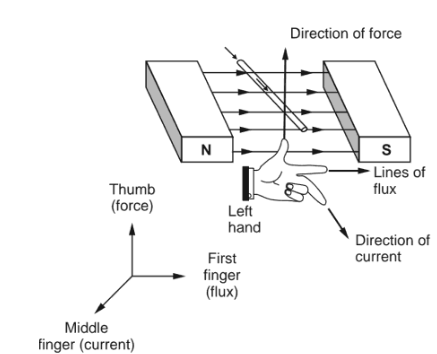# Recall the following Laws/Rules: Faraday’s Law of Electromagnetic Induction and Lenz’s Law

Faraday’s Law of Electromagnetic Induction is a fundamental law in physics that describes the relationship between a changing magnetic field and the induced electromotive force (EMF) in a conductor. The law states that an induced EMF is generated in a conductor when the magnetic flux linking the conductor changes over time.

The induced EMF can be calculated using Faraday’s law, which states that:

EMF = -dΦ/dt

where EMF is the induced electromotive force, Φ is the magnetic flux through the conductor, and t is time. The negative sign indicates that the direction of the induced EMF is such that it opposes the change in the magnetic flux.

Example: Consider a coil of wire placed in a changing magnetic field. As the magnetic field changes, the magnetic flux through the coil also changes, which in turn induces an EMF in the coil. If the magnetic flux through the coil increases, the induced EMF will be in the opposite direction to the current that would be produced if the same magnetic field were applied to a stationary coil.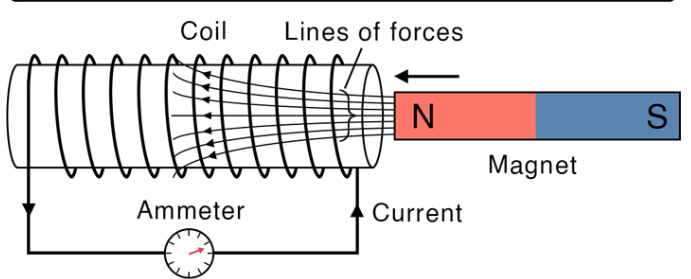Lenz’s Law is a consequence of Faraday’s Law and describes the direction of the induced EMF. Lenz’s law states that the direction of the induced EMF is such that it opposes the change that produced it.

Example: Consider a magnet being moved towards a stationary coil. As the magnet approaches the coil, the magnetic flux through the coil increases, inducing an EMF in the coil. According to Lenz’s law, the direction of the induced current will be such that it produces a magnetic field that opposes the motion of the magnet towards the coil. As a result, the coil will create a magnetic field in the opposite direction to the field created by the magnet, creating a force that repels the magnet.

In conclusion, Faraday’s Law of Electromagnetic Induction and Lenz’s Law are fundamental laws in physics that describe the relationship between a changing magnetic field and the induced electromotive force in a conductor. Faraday’s Law states that an induced EMF is generated in a conductor when the magnetic flux linking the conductor changes over time, while Lenz’s Law describes the direction of the induced EMF,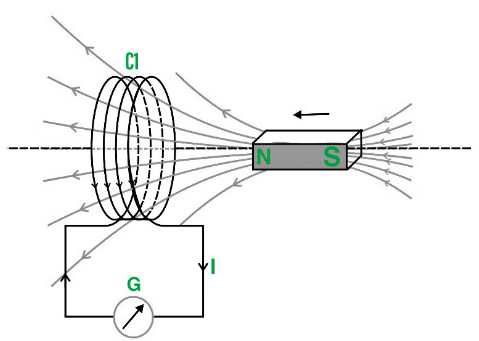which is such that it opposes the change that produced it.

# Recall the following Laws/Rules: Fleming’s Right-Hand Rule

Fleming’s Right-Hand Rule is a simple tool that helps us to determine the direction of a force acting on a current-carrying conductor in a magnetic field. This rule was developed by John Ambrose Fleming, a British scientist, in the late 19th century. It is an essential concept in understanding the working principles of electric motors, generators, and transformers.

Fleming’s Right-Hand Rule states that if we hold the thumb, index finger, and middle finger of our right hand perpendicular to each other and then point the index finger in the direction of the magnetic field, the middle finger in the direction of the current, then the thumb will point in the direction of the force acting on the conductor.

There are two variations of this rule, one for determining the direction of force acting on a straight conductor and the other for a current-carrying coil.

1. Fleming’s Right-Hand Rule for a Straight Conductor

Suppose we have a straight conductor carrying a current I placed in a magnetic field B. To determine the direction of the force acting on the conductor, we can use the following steps:

• Hold the thumb, index finger, and middle finger of the right hand perpendicular to each other.
• Point the index finger in the direction of the magnetic field B.
• Point the middle finger in the direction of the current I.
• The direction of the force acting on the conductor is given by the direction in which the thumb points.

For example, suppose we have a straight conductor carrying a current I = 5 A placed in a magnetic field B = 2 T pointing towards the east. By applying Fleming’s Right-Hand Rule, we can determine the direction of the force acting on the conductor. If we point the index finger towards the east, the middle finger towards the north (assuming the current is flowing from south to north), then the thumb will point towards the upward direction, indicating that the force acting on the conductor is upward.

1. Fleming’s Right-Hand Rule for a Current-Carrying Coil

Suppose we have a current-carrying coil placed in a magnetic field B. To determine the direction of the force acting on the coil, we can use the following steps:

• Hold the thumb, index finger, and middle finger of the right hand perpendicular to each other.
• Point the index finger in the direction of the magnetic field B.
• Point the middle finger in the direction of the current flowing through the coil.
• The direction of the force acting on the coil is given by the direction in which the thumb points.

For example, suppose we have a current-carrying coil placed in a magnetic field B pointing towards the north. The current flows in the clockwise direction when viewed from above. By applying Fleming’s Right-Hand Rule, we can determine the direction of the force acting on the coil. If we point the index finger towards the north, the middle finger in the direction of the current flow (clockwise), then the thumb will point towards the east direction, indicating that the force acting on the coil is towards the east.

# Recall the principle of Electromechanical Energy Conversion

Electromechanical energy conversion is the process of converting electrical energy into mechanical energy or vice versa. This process plays a vital role in the functioning of various electrical machines like motors, generators, transformers, etc. The principle of Electromechanical Energy Conversion is based on the interaction between the magnetic field and the electric current.

The principle of Electromechanical Energy Conversion can be explained by two important laws:

1. Faraday’s Law of Electromagnetic Induction

Faraday’s Law of Electromagnetic Induction states that whenever there is a change in the magnetic field, an emf (electromotive force) is induced in the conductor. This emf is proportional to the rate of change of magnetic flux linked with the conductor.

For example, suppose we have a conducting loop placed in a magnetic field. When the magnetic field is changed, an emf is induced in the loop. This emf can be calculated using the following equation:

emf = – dΦ/dt

where emf is the induced emf, Φ is the magnetic flux linked with the loop, and dt is the time taken for the change in the magnetic field.

1. Lorentz Force Law

Lorentz Force Law states that when a conductor carrying a current is placed in a magnetic field, a force is exerted on the conductor. The direction of the force is perpendicular to both the direction of the magnetic field and the direction of the current.

For example, suppose we have a straight conductor carrying a current I placed in a magnetic field B. According to Lorentz Force Law, a force F is exerted on the conductor, given by the following equation:

F = I * l * B * sinθ

where F is the force, I is the current flowing through the conductor, l is the length of the conductor, B is the magnetic field, and θ is the angle between the direction of the current and the direction of the magnetic field.

Based on these two laws, the principle of Electromechanical Energy Conversion can be explained as follows:

• In a motor, electrical energy is converted into mechanical energy. When a current-carrying conductor is placed in a magnetic field, a force is exerted on the conductor, which causes it to move. The direction of the force can be determined using the Fleming’s Right-Hand Rule. The movement of the conductor is used to rotate the shaft of the motor, which is connected to the load.
• In a generator, mechanical energy is converted into electrical energy. When a conductor is rotated in a magnetic field, an emf is induced in the conductor due to the change in the magnetic field. This induced emf can be used to power the electrical load.
• In a transformer, electrical energy is transferred from one circuit to another using the principle of mutual induction. When an alternating current flows through the primary winding of the transformer, it creates a changing magnetic field, which induces an emf in the secondary winding. This induced emf can be used to power the electrical load connected to the secondary winding.

Thus, the principle of Electromechanical Energy Conversion is an essential concept in understanding the working of various electrical machines, and it plays a crucial role in the generation, transmission, and distribution of electrical energy.

# Recall the concept of Energy and Co-energy

Energy and co energy are two important concepts in the field of electromechanical energy conversion. These concepts are used to analyze the energy flow in electrical machines like motors, generators, transformers, etc.

Energy:

Energy is the capacity to do work. In the context of electromechanical energy conversion, energy can be either electrical or mechanical. Electrical energy is the energy that is stored in an electrical system due to the presence of an electric charge. Mechanical energy is the energy that is stored in a mechanical system due to its position or motion.

In an electrical machine, electrical energy is converted into mechanical energy or vice versa. For example, in a motor, electrical energy is converted into mechanical energy, while in a generator, mechanical energy is converted into electrical energy. The amount of energy converted can be calculated using the following equation:

Energy = Power x Time

where Power is the rate at which energy is converted, and Time is the duration for which the energy is converted.

Co-energy:

Co-energy is the energy that is stored in the magnetic field of an electrical machine. When a current flows through a conductor, it creates a magnetic field around it. This magnetic field stores energy, which is known as co-energy.

The concept of co-energy is based on the principle of duality. According to this principle, energy can be stored in two forms: electric and magnetic. In an electrical machine, when electrical energy is converted into mechanical energy or vice versa, the magnetic field also changes. This change in the magnetic field causes a change in the co-energy stored in the magnetic field.

Co-energy is calculated using the following equation:

Co-energy = (1/2) x L x I2

where L is the inductance of the machine, and I is the current flowing through the machine.

The total energy stored in an electrical machine is the sum of the electrical energy, mechanical energy, and co-energy. This energy is conserved, which means that the total energy at any given time remains constant.

In summary, energy and co-energy are important concepts in the field of electromechanical energy conversion. Energy is the capacity to do work, and it can be either electrical or mechanical. Co-energy is the energy stored in the magnetic field of an electrical machine, and it is based on the principle of duality. The total energy stored in an electrical machine is the sum of the electrical energy, mechanical energy, and co-energy.

# Describe the Magnetic Energy stored in Singly Excited Systems

In a singly excited magnetic system, magnetic energy is stored in the magnetic field created by the excitation current flowing through the system. This magnetic energy is proportional to the square of the excitation current and the inductance of the system.

The magnetic energy stored in a singly excited system can be calculated using the formula:

Energy = 0.5 * L * I^2

where:

• Energy is the magnetic energy stored in the system (in joules).
• L is the inductance of the system (in henries).
• I is the excitation current flowing through the system (in amperes).

The magnetic energy stored in the system represents the potential energy stored in the magnetic field. This energy can be released and transferred back to the electrical system when the magnetic field collapses or when the excitation current is reduced or turned off. This energy transfer is often used in various applications, such as in magnetic storage devices, transformers, and inductive energy transfer systems.

It is important to note that the magnetic energy stored in a singly excited system is dependent on the inductance and the square of the excitation current. Therefore, increasing the inductance or the excitation current will result in higher magnetic energy stored in the system.

Conversely, reducing the inductance or the excitation current will decrease the magnetic energy stored.

Proper management and control of the excitation current and inductance are crucial to ensure the efficient and safe operation of singly excited magnetic systems. This includes considerations such as limiting the maximum excitation current to prevent saturation of the magnetic materials and implementing appropriate protection measures to handle the release of the stored magnetic energy.

# Describe the Torque developed in Doubly Excited Systems

Doubly excited systems are those in which both the electrical and magnetic fields are present and are coupled with each other. In such systems, the torque developed is a function of both the electrical and magnetic fields. The torque developed in a doubly excited system can be calculated using the following formula:

T = Kφ1φ2

where T is the torque developed, K is a constant, φ1 is the flux produced by the electrical field, and φ2 is the flux produced by the magnetic field.

The flux produced by the electrical field is proportional to the current flowing through the electrical circuit, while the flux produced by the magnetic field is proportional to the current flowing through the magnetic circuit. Therefore, the torque developed in a doubly excited system is a function of both the electrical and magnetic currents.

The torque developed in a doubly excited system can be used for various purposes, such as in electric motors, generators, and actuators. For example, in an electric motor, the torque developed is used to convert electrical energy into mechanical energy. The electrical energy is supplied to the motor through the electrical circuit, while the magnetic circuit produces the magnetic field that produces the torque.

In a generator, the torque developed is used to convert mechanical energy into electrical energy. The mechanical energy is supplied to the generator through a rotating shaft, which produces the magnetic field that produces the electrical energy.

In an actuator, the torque developed is used to produce a mechanical output, such as moving a valve or a damper. The electrical circuit provides the current that produces the electrical field, while the magnetic circuit produces the magnetic field that produces the torque.

In conclusion, the torque developed in a doubly excited system is a function of both the electrical and magnetic fields. The torque can be used for various purposes, such as in electric motors, generators, and actuators. The electrical and magnetic fields are coupled with each other, and the torque developed depends on the current flowing through both the circuits.